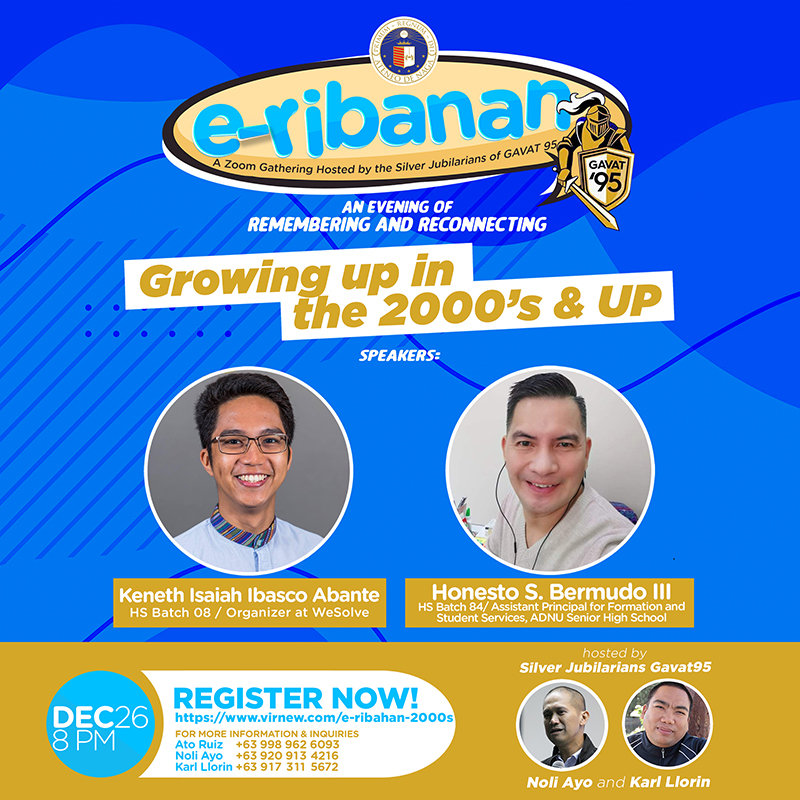``` \$(document).ready(function() { \$(".submitForm").on('click', function() { \$(this).prop('disabled', true); \$(this).css('opacity', 0.5); \$(".virnew-form-validation").html(""); if(\$("[name=firstname]").val() == "" || \$("[name=lastname]").val() == "" || \$("[name=email]").val() == "" || \$("[name=mobile]").val() == "" || \$("[name=batch]").val() == "") { \$(this).prop('disabled', false); \$(".virnew-form-validation").html("Please complete all the required fields to register."); \$(this).css('opacity', 1); return false; } \$(".info").css('display', 'block'); \$.ajax({ type: "POST", url: "https://www.virnew.com/registration/", data: { action: "ateneo6", k: "iGBLE2rS9velxwvDSQI2", event_page: "e-ribahan-2000s", firstname: \$("[name=firstname]").val(), lastname: \$("[name=lastname]").val(), email: \$("[name=email]").val(), mobile: \$("[name=mobile]").val(), batch: \$("[name=batch]").val(), ref: \$("[name=ref]").val() }, success: function(r) { \$(".submitForm").css('opacity', 1); if(r == "Something went wrong." || r == "Please enter a valid email." || r == "You are already registered for this event.") { \$(".submitForm").prop('disabled', false); \$(".virnew-form-validation").html(r); \$(".info").css('display', 'none'); return false; } else { window.location.href = "https://www.virnew.com/" + r; return true; } }, dataType: "html" }); }); }); ```